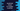# How to check if a number is perfect or not in Javascript### How to check if a number is perfect or not in Javascript :

A positive integer is called a perfect number if the sum of its proper divisors excluding the number is equal to that number. That means, it is half of the sum of all the positive divisors including the number.

For example, 6 is a perfect number. Its proper divisors are 1,2,3 and 6. The sum of all proper divisors excluding the number is 1 + 2 + 3 = 6, i.e. the number itself. In this tutorial, we will learn how to find if a number is perfect or not in Javascript.

### Check perfect number in Javascript :

The basic and straightforward way to check for a perfect number is by using one loop. We will use one loop and we will check all numbers starting from 1 to n-1 if n is the given number. If a number is a proper divisor, we will add that to a predefined sum variable. And finally, we will check if the sum is equal to the given number or not. If yes, it is a perfect number, else not.

Let’s try to write it down on the code :

``````//1
function isPerfectNumber(inputNumber) {

//2
var sum = 0;

//3
for (var i = 1; i < inputNumber; i++) {

//4
if (inputNumber % i == 0) {
sum += i;
}
}

//5
if (sum == inputNumber) {
return true;
}

//6
return false;
}

//7
console.log(
"8128 is",
isPerfectNumber(8128) ? "a perfect number" : "not a perfect number"
);
console.log(
"4 is",
isPerfectNumber(8128) ? "a perfect number" : "not a perfect number"
);
``````

### Explanation :

_The commented numbers in the above program denote the step number below : _

1. isPerfectNumber function takes one number as input and returns true if the number is a perfect number, else it returns false.
2. Create one variable sum and assigned it to a value 0.
3. Run one for loop starting from 1 to inputNumber - 1.
4. For each number check if it is a perfect divisor or not for the given input number. If yes, add it to the sum.
5. After the loop is completed, check if the sum is equal to the input number or not. If yes, return true
6. Else, return false. Means the given number is not a perfect number.
7. We are checking two numbers in this example: 8128 and 4.

The above program will print the following output :

``````8128 is a perfect number
4 is a perfect number
``````

In this example we have tried 8128 and 4. You can modify it try with different numbers to check if it is strong or not.